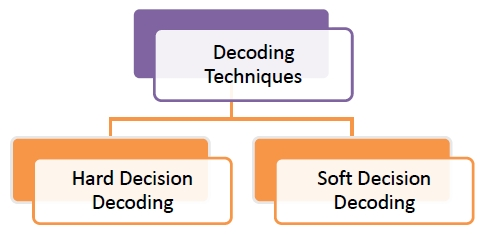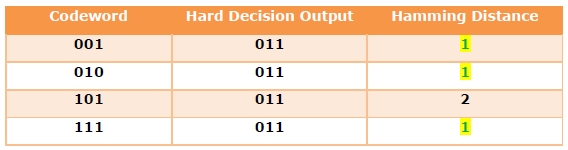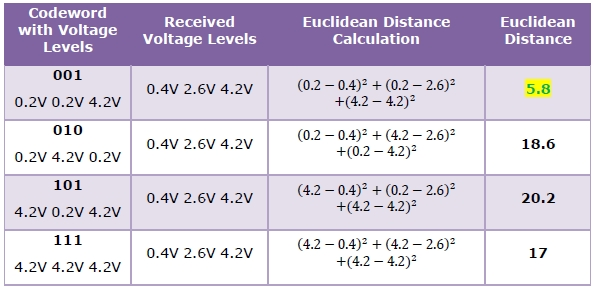# Hard and Soft Decision Decoding

A challenging task in error correction is decoding the codewords that have been received via noisy channels. Before data is transmitted, the sender adds redundant bits or parity bits to the message forming codewords. The codewords are then transmitted via computer networks. The receiver checks the incoming codewords and performs the decoding or error correction process to retrieve the original data.

If there are no errors, i.e. the codewords find an exact matching, then it is easy to decode the data by eliminating the parity bits. However, if a match is not found, then more complex decoding mechanisms are adopted.

The two categories of decoding techniques are −## Hard Decision Decoding

Hard decision decoding takes a stream of bits or a block of bits from the threshold stage of receiver, and decodes each bit by considering it as definitely 1 or 0. It samples the received pulses and compares their voltages to threshold values. If a voltage is greater than the threshold value, it is decoded as 1 and otherwise decoded as 0. The decoding is done irrespective of how close the voltage is to the threshold.

## Soft Decision Decoding

Soft decision decoding is a class of algorithms that takes a stream of bits or a block of bits and decodes them by considering a range of possible values that it may take. It considers the reliability of each received pulse to form better estimates of input data.

Soft-decision decoders are often used in Viterbi decoders that are used for decoding convolutional codes.

## Example

Let us consider that the valid set of codewords that are sent by the source encoder is −

• 001
• 010
• 101
• 111

Suppose that the bit ‘0’ is encoded as 0.2V and ‘1’ bit is encoded as 4.2V. If the sender wants to send the codeword 001, it sends pulses of voltages 0.2V, 0.2V, 4.2V.

Suppose that this message is attenuated during transmission and the voltages received at destination are 0.4V, 2.6V, 4.2V.

The following two cases show decoding by hard decision method and soft decision methods.

## Hard Decision Decoding

Let us consider that the threshold voltage chosen by the hard decision decoder is 2.2V. Any voltage received above 2.2V is considered as 1 bit and any voltage received below 2.2V is considered 0 bit.

So, the hard decision decoder considers the incoming voltages 0.4V, 2.6V, 4.2V as 011.

Since 011 is not a valid codeword, the hard decision decoder compares the Hamming distances of this data with the set of valid codewords and finds the minimum Hamming distance.In this case, there are 3 codewords with the minimum Hamming distance of 1. The decoder chooses any of them randomly with a probability of 1/3.

Thus it can be seen that hard decision decoding has scope for ambiguity.

## Soft Decision Decoding

There are various soft decision decoding algorithms. Let us consider that in this situation, the soft decision decoder calculates the Euclidean distances between the received voltages and the voltages of the codewords received. It then finds the minimum Euclidean distance and selects the corresponding codeword.It can be seen that the minimum Euclidean distance corresponds to the codeword 001, which has been actually sent by the source.

Thus soft-decision decoding technique provides better error correction capability than hard decision decoding.

Updated on: 27-Jun-2020

5K+ Views